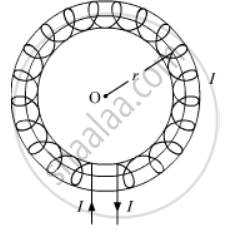# An Iron Ring of Relative Permeability µR Has Windings of Insulated Copper Wire of N Turns per Meter. When the Current in the Windings is I, Find the Expression for the Magnetic Field in the Ring. - Physics

#### Question

An iron ring of relative permeability µr has windings of insulated copper wire of n turns per meter. When the current in the windings is I, find the expression for the magnetic field in the ring.

#### SolutionAn iron ring of relative permeability µr having windings of insulated copper wire of n turns per meter can be considered as a toroid. Consider a toroidal solenoid with centre O as shown.

Suppose that
r = average radius of the toroid
I = current through the solenoid
To determine the magnetic field produced at the centre along the axis of the toroid due to current I, we imagine an Amperian loop of radius r and traverse it in the clockwise direction.
According to Ampere's circuital law, we have:

ointvecB.vec(dl) = mu_rI

The total current flowing through the toroid is NI, where N is the total number of turns.

ointvecB.vec(dl) = mu_r(NI) ......(1)

Now vecB and vec(dl) are in the same direction

oint vecB.vec(dl) = B.ointdl

=> ointvecb.vec(dl) = B(2pir) ....(2)

Comparing equations (1) and (2), we get:

B(2pir) = mu_rNI

=> B = (mu_rNI)/(2pir)

Now it is given that n is the number of turns per unit length, then

n = N/(2pir)

∴B = mu_0nI which is the expression for the magnetic field in the ring.

Is there an error in this question or solution?